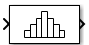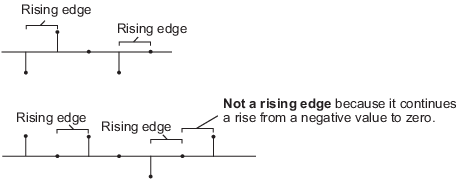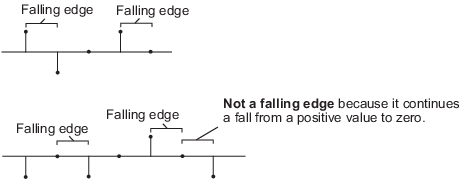# Histogram

Histogram of input or sequence of inputs

• Library:
• DSP System Toolbox / Statistics

•## Description

The Histogram block computes the frequency distribution of the input elements along each column or along the entire input. You can specify the dimension using the Find the histogram over parameter. The block distributes the input elements based on their value into a number of discrete bins specified by the Number of bins parameter. For more details, see Algorithms.

When the input data is real, the bin boundaries are cast into the double data type. When the input data is complex, bin boundaries for double-precision inputs are cast into double, and bin boundaries for integer inputs are cast into double and squared. For an example, see Compute the Histogram of Real and Complex Data.

To track the frequency distribution of inputs over a period of time, select the Running histogram parameter.

When the input is complex, the block sorts the elements by their magnitude.

## Ports

### Input

expand all

The block accepts real-valued or complex-valued multichannel and multidimensional inputs. The input data type must be double precision, single precision, integer, or fixed point, with power-of-two slope and zero bias.

This port is unnamed until you select the Running histogram parameter and set the Reset port parameter to any option other than `None`.

Data Types: `single` | `double` | `int8` | `int16` | `int32` | `int64` | `uint8` | `uint16` | `uint32` | `uint64` | `fixed point`
Complex Number Support: Yes

Specify the reset event that causes the block to reset the running histogram. The reset signal and the input data signal must have the same rate.

#### Dependencies

To enable this port, select the Running histogram parameter, and set the Reset port parameter to any option other than `None`.

Data Types: `single` | `double` | `int8` | `int16` | `int32` | `uint8` | `uint16` | `uint32` | `Boolean`

### Output

expand all

Outputs the histogram data as a vector, matrix, or array. The output primarily depends on the settings of the Running histogram and Find the histogram over parameters.

For example, consider a two-dimensional input signal of size M-by-N.

When you clear the Running histogram parameter, the block computes the frequency distribution in each column of input or along the entire input. When you set Find the histogram over to:

• `Each column` — The block computes a histogram for each column of the input. The output is an n-by-N matrix, where n is the Number of bins. The jth column of the output matrix contains the histogram for the data in the jth column of the M-by-N input matrix.

• `Entire input` — The block computes a histogram for the entire input vector, matrix, or N-D array. The output is an n-by-1 vector, where n is the Number of bins.

When you select the Running histogram parameter, the block computes the frequency distribution of all inputs over a period of time. In this case, when you set Find the histogram over to:

• `Each column` — The block computes a running histogram for each column of the input. The output is an n-by-N matrix, where n is the Number of bins. The jth column of the output matrix contains the running histogram for the data in the jth column of the M-by-N matrix input.

• `Entire input` — The block computes the running histogram for the entire input vector, matrix, or N-D array. The output is an n-by-1 vector, where n is the Number of bins.

The output data type is `uint32` when the input has a data type other than `single` or `double`. The largest number that can be represented by `uint32` is `232-1`. If the range of any input exceeds this value, the block wraps the value back to `0`.

Data Types: `single` | `double` | `uint32`

## Parameters

expand all

### Main Tab

Specify the lower boundary of the lowest-valued bin as a real scalar. This parameter does not accept `NaN` or `Inf`. If the input has a value less than Lower limit of histogram, the block places this element in the lowest-valued bin.

Specify the upper boundary of the highest-valued bin as a real scalar. This parameter does not accept `NaN` or `Inf`. If the input has a value greater than Upper limit of histogram, the block places this element in the highest-valued bin.

Specify the number of histogram bins as a positive integer.

Specify whether the histogram is computed over each column or the entire input. The options for this parameter are affected by the setting of the Running histogram parameter.

When you clear the Running histogram parameter, and you set Find the histogram over parameter to:

• `Each column` — The block computes the histogram over each column.

• `Entire input` — The block outputs the histogram over the entire input.

When you select the Running histogram parameter, and you set Find the histogram over parameter to:

• `Each column` — The block computes the running histogram over each column.

• `Entire input` — The block outputs the running histogram over the entire input.

When you select this parameter, the block output, y, is normalized. In mathematical terms, `sum(y) = 1`. This parameter does not apply for fixed-point input signals.

When you select this parameter, the block tracks the frequency distribution of inputs over a period of time.

The block resets the running histogram and empties all the bins whenever a reset event is detected at the optional Rst port. The reset sample time must equal the input sample time.

Use this parameter to specify the reset event:

• `Non-zero sample` — Triggers a reset operation at each sample time when the Rst input is not zero.

• `None` — Disables the Rst port.

• `Rising edge` — Triggers a reset operation when the Rst input does one of the following:

• Rises from a negative value to either a positive value or zero.

• Rises from zero to a positive value, where the rise is not a continuation of a rise from a negative value to zero.• `Falling edge` — Triggers a reset operation when the Rst input does one of the following:

• Falls from a positive value to a negative value or zero.

• Falls from zero to a negative value, where the fall is not a continuation of a fall from a positive value to zero.• `Either edge` — Triggers a reset operation when the Rst input is a `Rising edge` or `Falling edge`.

Note

When running simulations in the Simulink® multitasking mode, reset signals have a one-sample latency. When the block detects a reset event, there is a one-sample delay at the reset port rate before the block applies the reset. For more information on latency and the Simulink tasking modes, see Excess Algorithmic Delay (Tasking Latency) and Time-Based Scheduling and Code Generation (Simulink Coder).

#### Dependencies

To enable this parameter, select the Running histogram parameter.

### Data Types Tab

Note

To use these parameters, the data input must be complex and fixed point. For all other inputs, the parameters on the Data Types tab are ignored.

Specify the rounding mode for fixed-point operations. For more details, see rounding mode.

When you select this parameter, the block saturates the result of its fixed-point operation. When you clear this parameter, the block wraps the result of its fixed-point operation. For details on `saturate` and `wrap`, see overflow mode for fixed-point operations.

The squares of the real and imaginary parts of the complex input are stored in the Product output data type.

You can set this parameter to:

• `Inherit: Same as input` — The product output data type is the same as the input data type.

• `fixdt([],16,0)` — The product output data type is an autosigned, binary-point, scaled, fixed-point data type with a word length of 16 bits and a fraction length of 0.

Alternatively, you can set the Product output data type by using the Data Type Assistant. To use the assistant, click the button.

For more information on the data type assistant, see Specify Data Types Using Data Type Assistant (Simulink).

The result of the sum of the squares of the real and imaginary parts of the complex input are stored in the Accumulator data type.

You can set this parameter to:

• `Inherit: Same as input` — The accumulator data type is the same as the input data type.

• `Inherit: Same as product output` — The accumulator data type is the same as the product output data type.

• `fixdt([],16,0)` — The accumulator data type is an autosigned, binary-point, scaled, fixed-point data type with a word length of 16 bits and a fraction length of 0.

Alternatively, you can set the Accumulator data type by using the Data Type Assistant. To use the assistant, click the button.

For more information on the data type assistant, see Specify Data Types Using Data Type Assistant (Simulink).

Select this parameter to prevent the fixed-point tools from overriding the data types you specify on the block.

## Block Characteristics

 Data Types `double` | `fixed point` | `integer` | `single` Direct Feedthrough `no` Multidimensional Signals `no` Variable-Size Signals `no` Zero-Crossing Detection `no`

expand all

## Version History

Introduced before R2006a